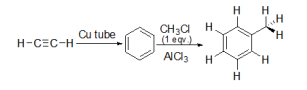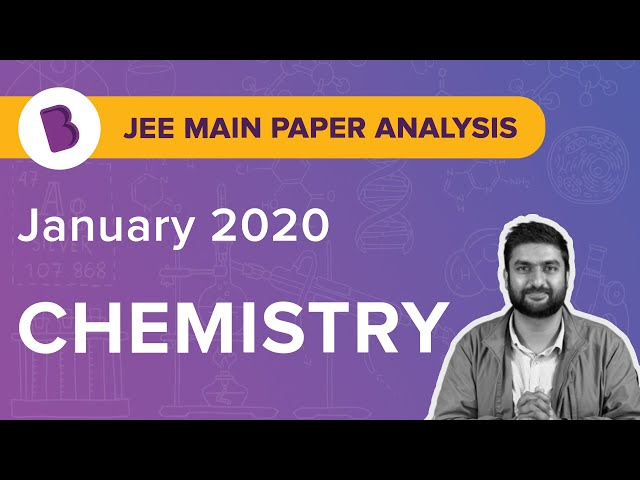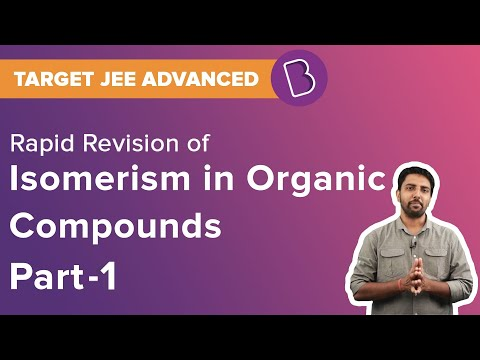Win up to 100% scholarship on Aakash BYJU'S JEE/NEET courses with ABNAT Win up to 100% scholarship on Aakash BYJU'S JEE/NEET courses with ABNAT

# JEE Main 2020 Paper with Solutions Shift 2 8th Jan Chemistry

Students aspiring to join some of the prestigious institutions in the country must get a good score in JEE. The students should definitely solve previous years’ question papers to crack the examination. All the questions from JEE Main 2020 Chemistry (January 8, Shift 2) are solved here. Learn these questions to excel in the test.
January 8 Shift 2 – Chemistry
1. Arrange the following bonds according to their average bond energies in descending order:

C-Cl, C-Br, C-F, C-I

a. C-Cl > C-Br > C-I > C-F
b. C-Br>C-I>C-Cl>C-F
c. C-I>C-Br>C-Cl>C-F
d. C-F>C-Cl>C-Br>C-I

In C − F there is 2p-2p overlapping involved, in C − Cl the overlapping involved is 2p-3p whereas for C − Br and C − I the overlapping involved are 2p-4p and 2p-5p, respectively. The bond length for the various type of overlapping can be given as:

2p-2p < 2p-3p < 2p-4p < 2p-5p.

As we know that Bond energy α (1/Bond length)

The order of bond energy comes out: C-F > C-Cl > C-Br > C-I

2. The radius of second Bohr orbit, in terms of the Bohr radius, 𝑎0 , in Li2+ is:
a. 2a0/3
b. 4a0/9
c. 4a0/3
d. 2a0/9

The formula for Bohr’s radius for any one electron species is: r = a0n2/ z

for Li2+

: r = a022/3

= 4a0/3

3. A metal (A) on heating in nitrogen gas gives compound B. B on treatment with 𝐻2𝑂 gives a colourless gas which when passed through 𝐶𝑢𝑆𝑂4 solution gives a dark blue-violet coloured solution. A and B respectively, are :
a. Na and Na3N
b. Mg andMg3N2
c. Mg, Mg(NO3)2
d. Na, NaNO3

As it is provided in the question that nitride is being formed so the option c and d can be eliminated. Amongst Mg and Na we already know that Mg can only form nitride so the correct choice is option a.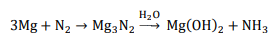4. The correct order of the calculated spin-only magnetic moments of complexes A to D is:

A. 𝑁i(𝐶𝑂)4

B. [𝑁𝑖(𝐻2𝑂)6]2+

C. 𝑁𝑎2[𝑁𝑖(𝐶𝑁)4]

D. 𝑃𝑑𝐶𝑙2(𝑃𝑃ℎ3)2

a. (C) < (D) < (B) < (A)
b. (A) ≈ (C) ≈ (D) < (B)
c. (A) ≈ (C)<(B) ≈ (D)
d. (C) ≈ (D) < (B) < (A)

[Pd(PPh3)2Cl2] : Here Pd is in +2 oxidation state and configuration of Pd 2+ is [Kr]4d8 . As the CFSE value for Pd is very high so all the electrons will be paired and hence magnetic moment for this complex will be zero.

[Ni(CO)4] : Here Ni is in 0 oxidation state and configuration of Ni is [Ar]3d84s2 . As here the ligand is carbonyl which is a strong field ligand, all the electrons will be paired and hence magnetic moment for this complex will be zero.

[Ni(CN)4]2- : Here Ni is in +2 oxidation state and configuration of Ni2+ is [Ar]3d8 . As here the ligand is cyanide which is a strong field ligand, all the electrons will be paired and hence magnetic moment for this complex will be zero.

[Ni(H2O)6]2+ : Here Ni is in +2 oxidation state and configuration of Ni2+ is [Ar]3d8 . As here the ligand is water which is a weak field ligand, the electrons will not be paired and there are two unpaired electrons in this complex hence magnetic moment for this complex will be √8 BM.

So the order of magnetic moment is A=B=C<D.

5. Hydrogen has three isotopes (A), (B) and (C). If the number of neutron(s) in (A), (B) and (C) respectively, are (x), (y) and (z), the sum of (x), (y) and (z) is:
a. 4
b. 1
c. 3
d. 2

Number of neutrons in protium = 0

Number of neutrons in deuterium = 1

Number of neutrons in tritium = 2

So, total number of neutrons = 3

6. Consider the following plots of rate constant versus 1/𝑇 for four different reactions. Which of the following orders is correct for the activation energies of these reactions?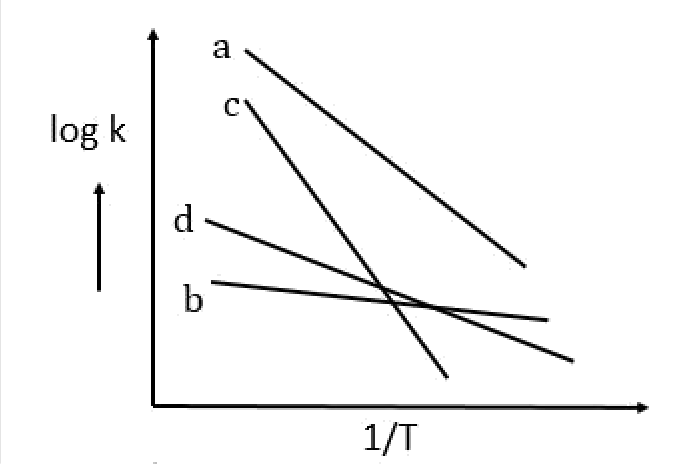a. Ea > Ec > Ed > Eb
b. Ec > Ea > Ed > Eb
c. Eb > Ed > Ec > Ea
d. E > Ea > Ed > Ec

To avoid confusion, in this question we’ll be denoting activation energy by Ex

K = Ae−Ex/RT

log K = log A – (Ex/2.303RT) —–(1)

Here, the graph given in the question is of a straight line and we know that the equation of straight

line is

y= mx + c —-(2)

Comparing equation 1 with 2 we get,

Slope = −Ex/2.303R

So, from the graph we can conclude that the line with the most negative slope will have the

maximum activation energy value.

Ec > Ea > Ed > Eb

7. Which of the following compounds is likely to show both Frenkel and Schottky defects in its

crystalline form?
a. ZnS
b. CsCl
c. KBr
d. AgBr
The radius ratio for AgBr is intermediate. Thus, it shows both Frenkel and Schottky defects.

8. White phosphorus on reaction with concentrated NaOH solution in an inert atmosphere of 𝐶𝑂2 gives phosphine and compound (X). (X) on acidification with HCl gives compound (Y). The basicity of compound (Y) is:
a. 4
b. 2
c. 3
d. 1

Here the product B which is mentioned in the question is H3PO2. The structure of H3PO2 can be given as: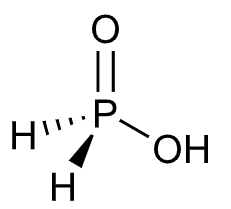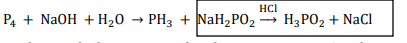As only 1 Hydrogen atom is attached to the oxygen, its basicity is one.

9. Among the reactions (a) – (d), the reaction(s) that does/do not occur in the blast furnace during the extraction of iron is/are:

A. CaO + SiO2→ CaSiO3

B. 3Fe2O3 + CO → 2Fe3O4 + CO2

C. FeO +SiO2 →FeSiO3

D. FeO → Fe + (1/2)O2

a. A
b. D
c. C and D
d. A and D

In metallurgy of iron, CaO is used as flux which is used to remove the impurities of SiO2,

CaO + SiO2→ CaSiO3 .

Also here Fe2O3 is reduced by CO to Fe3O4 which is further reduced to FeO which is further reduced

to Fe.

3Fe2O3 + CO → 2Fe3O4 + CO2

Fe3O4 + CO → 3FeO + CO2

FeO + CO → Fe + CO2

10. The increasing order of the atomic radii of the following elements is:

A. C

B. O

C. F

D. Cl

E. Br

a. B<C<D<A<E
b. C<B<A<D<E
c. A<B<C<D<E
d. D<C<B<A<E l

Across the period size decreases, so the order that follows is: C > O >N> F

Down the group size increases and the order is: Br > Cl > F

Change in size down the group is much more significant as compared to across the period.

So, the overall order of radius of elements is: Br > Cl > C > O > F.

11. Among (a) – (d), the complexes that can display geometrical isomerism are:

A. [Pt(NH3)3Cl]+

B. [Pt(NH3)Cl5]

C. [Pt(NH3)2Cl(NO2)]

D. [Pt(NH3)4ClBr] 2+

a. A and B
b. D and A
c. C and D
d. B and C
The complexes of type Ma4bc and Ma2b2 can show geometrical isomerism provided Ma2b2 is square planar. The compound given in B is Ma4bc type and compound in D is Ma2b2 type also in D, Ni is surrounded with strong field ligands which will result in dsp 2 hybridisation and hence square planar geometry.

12. For the following Assertion and Reason, the correct option is:

Assertion: The pH of water increases with increase in temperature.

Reason: The dissociation of water into H+ and OH an exothermic reaction.

a. Both assertion and reason are false.
b. Assertion is not true, but reason is true.
c. Both assertion and reason are true and the reason is the correct explanation for the assertion.
d. Both assertion and reason are true, but the reason is not the correct explanation for the assertion.

H2O → H+ + OH is an endothermic process.

On increasing the temperature the value of Kw

increases which will result in decrease in pKw . So we can say that pH of water will decrease on

increasing temperature because pH for water =1/2pKw.

13. For the following Assertion and Reason, the correct option is:

Assertion: For hydrogenation reactions, the catalytic activity increases from group-5 to group11 metals with maximum activity shown by group 7-9 elements

Reason: The reactants are most strongly adsorbed on group 7-9 elements

a. Both assertion and reason are false.
b. The assertion is true, but the reason is false.
c. Both assertion and reason are true, but the reason is not the correct explanation of assertion
d. Both assertion and reason are true and the reason is the correct explanation of assertion

Group 7-9 elements of the periodic table show variable valencies so they have maximum activity because of the increase in adsorption rate.

14. The major product of the following reactions is: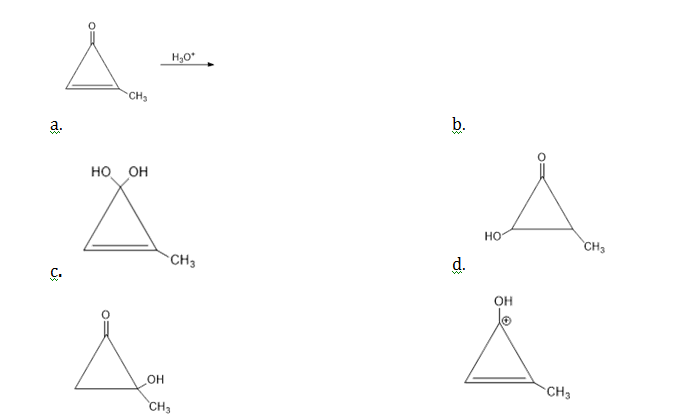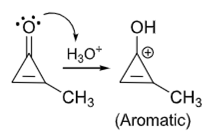15. Find The major product [B] of the following sequence of reactions is: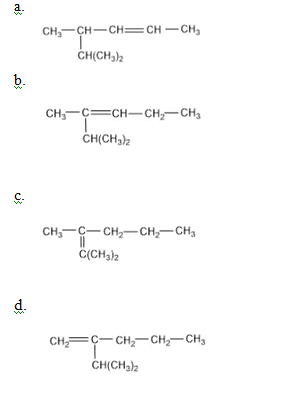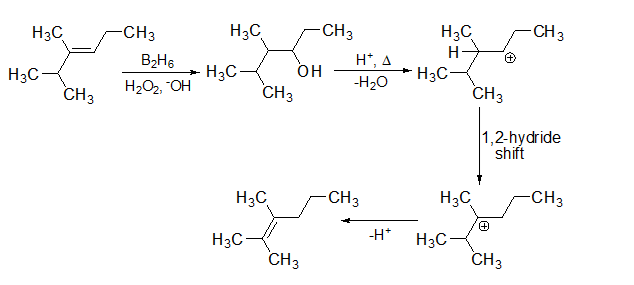16. Among the following compounds A and B with molecular formula C9H18O3, A is having higher boiling point than B. The possible structures of A and B are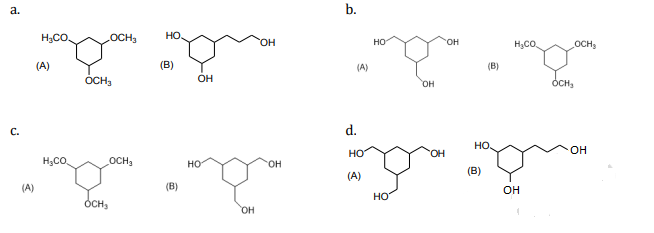In option b compound A has extensive inter-molecular hydrogen bonding because of the 3 −OH groups while in compound B there are −OCH3 groups present and no inter-molecular hydrogen bonding is possible.

17. Kjeldahl’s method cannot be used to estimate nitrogen for which of the following compounds?
a. 𝐶𝐻3𝐶𝐻2 − 𝐶 ≡ 𝑁
b. 𝐶6𝐻5𝑁𝐻2
c. 𝐶6𝐻5𝑁𝑂2
d.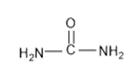Kjeldahl method cannot be used for the estimation of nitrogen in the compounds in which nitrogen is involved in nitro, diazo groups or is present in the ring, as nitrogen atom can’t be converted to ammonium sulphate under the reaction conditions.

18. An unsaturated hydrocarbon absorbs two hydrogen molecules on catalytic hydrogenation, and also gives following reaction;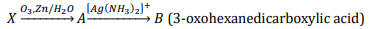𝑋 will be: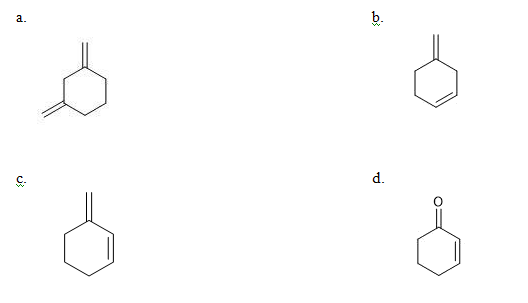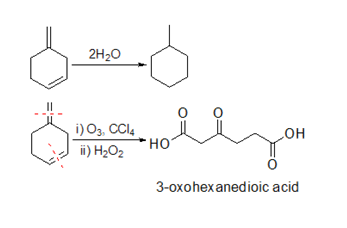19: Preparation of Bakelite proceeds via reactions:
a. Electrophilic substitution and dehydration.
c. Condensation and elimination

Bakelite is a condensation polymer of phenol and formaldehyde.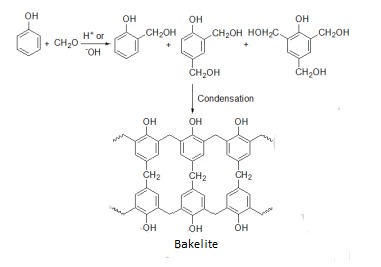20. Two monomers of maltose are:
a. alpha-D-Glucose and alpha-D-Galactose
b. alpha-D-Glucose and alpha-D-Glucose
c. alpha-D-Glucose and alpha-D-Fructose
d. alpha-D-Glucose and alpha-D-Glucose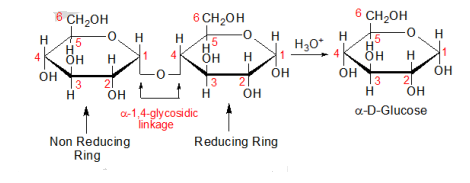Maltose is formed by the glycosidic linkage between C-1 of one alpha -D-Glucose unit to the C-4 of another alpha -D-Glucose.

21: At constant volume, 4 mol of an ideal gas when heated from 300 K to 500 K changes its internal energy by 5000 J. The molar heat capacity at constant volume is _____.

∆U = nCv∆T

5000 = 4 × Cv (500 – 300)

Cv = 6.25 JK–1 mol–1

22: For an electrochemical cell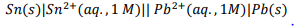The ratio [Sn2+]/[Pb2+] when this cell attains equilibrium is ______.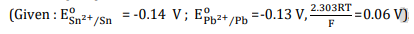Anodic half: Sn → Sn 2+ + 2e

Cathodic half: Pb 2+ + 2e → Pb

Net reaction: Sn + Pb 2+ → Pb + Sn 2+

E0cell = E0cathode − E0anode

E0cell = 0.01 V

Ecell = E0cell− (0.06/2) logQ

At equilibrium state Ecell = 0

So,

0 = 0.01 – (0.06/2)log [Sn 2+]/[Pb 2+]

0.01 = (0.06/2)log [Sn 2+]/[Pb 2+]

log [Sn 2+]/[Pb 2+] =1/3

[Sn 2+]/[Pb 2+] = 2.154

23. NaClO3 is used, even in spacecrafts, to produce O2. The daily consumption of pure O2 by a person is 492 L at 1 atm, 300 K. How much amount of NaClO3, in grams, is required to produce O2 for the daily consumption of a person at 1 atm, 300 K ?

NaClO3(s) + Fe(s) → O2(g) + FeO(s) + NaCl(s)

R = 0.082 L atm mol–1 K–1

Mol of NaClO3 = mol of O2

Mol of O2 =PV/RT =(1×492)/(0.082×300)= 20 mol

Molar mass of NaClO3 is 106.5

So, mass = 20 x106.5 = 2130 g = 2.13 kg

24. Complexes [ML5] of metals Ni and Fe have ideal square pyramidal and trigonal bipyramidal and geometries, respectively. The sum of the 900, 1200 and 1800 L-M-L angles in the two complexes is ______.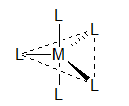For trigonal bipyramidal geometry

Total number of 1800 L-M-L bond angles = 1

Total number of 900 L-M-L bond angles = 6

Total number of 1200 L-M-L bond angles = 3

Total = 10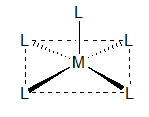For square pyramidal geometry

Total number of 1800 L-M-L bond angles = 2

Total number of 900 L-M-L bond angles = 8

Total number of 1200 L-M-L bond angles = 0

Total = 10

Total for both the structures = 20

25: In the following sequence of reactions, the maximum number of atoms present in molecule ‘C’ in

one plane is _____.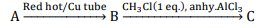(Where A is a lowest molecular weight alkyne).